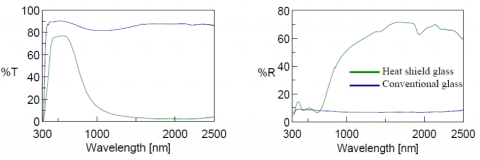# Evaluation of Solar Heat Gain Coefficient and Transmittance, Reflectance & Emittance Measurement of Flat Glass by UV-Visible/NIR Spectroscopy

September 14, 2017

## Introduction

Heat shield glass is typically made from eco-friendly materials that help in the prevention of global warming and reduction in energy costs. Testing methods for the evaluation of heat shield glass is stipulated by JIS R3016 and require visible transmittance, visible reflectance, solar transmittance, solar reflectance, and emittance measurements. From these measurements, the solar heat gain coefficient can be calculated, which is defined as the fraction of incident solar radiation admitted through a window.

This application note shows how to obtain and evaluate transmittance/reflectance spectra of a flat glasses using an integrating sphere and calculate the solar radiation absorbance using the Solar Transmittance/Reflectance Visible Light Transmittance/Reflectance program.

## Experimental

 Measurement Conditions Measurement Range 2500-300nm Data Pitch 0.5 nm UV/Vis Bandwidth 5.0 nm NIR Bandwidth 25.0 nm Scan Speed 400 nm/min Response Fast

## Keywords

V-670, UV-Visible/NIR, ISN-723 Integrating sphere, VWST-774 Solar Transmittance/Reflectance Visible Light Transmittance/Reflectance program, solar heat gain

## Results

Spectra of both heat shield glass and conventional glass were measured and are shown in Figure 1.Figure 1. Transmittance (left) and reflectance (right) spectra of heat shield glass (green) and conventional glass (blue).

The solar radiation results of the heat shield and conventional glass were calculated using the Solar Transmittance/Reflectance Visible Light Transmittance/Reflectance program and are shown in Figure 2 and Table 1.